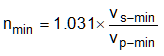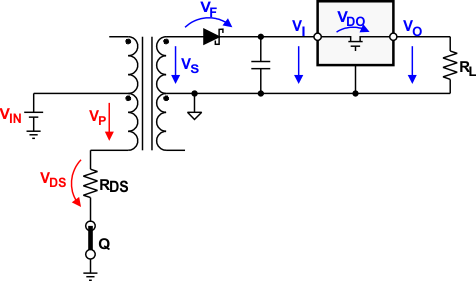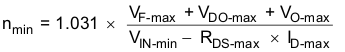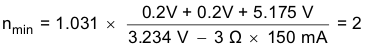SLLSEF3C June   2013  – March 2021

PRODUCTION DATA

1. Features
2. Applications
3. Description
4. Revision History
5. Pin Configuration and Functions
6. Specifications
7. Parameter Measurement Information
8. Detailed Description
1. 8.1 Overview
2. 8.2 Functional Block Diagram
3. 8.3 Feature Description
4. 8.4 Device Functional Modes
9. Application and Implementation
1. 9.1 Application Information
2. 9.2 Typical Application
1. 9.2.1 Design Requirements
2. 9.2.2 Detailed Design Procedure
3. 9.2.3 Application Curve
4. 9.2.4 Higher Output Voltage Designs
5. 9.2.5 Application Circuits
10. 10Power Supply Recommendations
11. 11Layout
12. 12Device and Documentation Support
13. 13Mechanical, Packaging, and Orderable Information

#### パッケージ・オプション

• DBV|5
##### 9.2.2.5.2 Turns Ratio Estimate

Assume the rectifier diodes and linear regulator has been selected. Also, it has been determined that the transformer choosen must have a V-t product of at least 11 Vμs. However, before searching the manufacturer websites for a suitable transformer, the user still needs to know its minimum turns ratio that allows the push-pull converter to operate flawlessly over the specified current and temperature range. This minimum transformation ratio is expressed through the ratio of minimum secondary to minimum primary voltage multiplied by a correction factor that takes the transformer’s typical efficiency of 97% into account.

Equation 5.VS-min must be large enough to allow for a maximum voltage drop, VF-max, across the rectifier diode and still provide sufficient input voltage for the regulator to remain in regulation. From the LDO SELECTION section, this minimum input voltage is known and by adding VF-max gives the minimum secondary voltage with:

Equation 6. VS-min = VF-max + VDO-max + VO-maxFigure 9-5 Establishing the Required Minimum Turns Ratio Through Nmin = 1.031 × VS-min / VP-min

Then calculating the available minimum primary voltage, VP-min, involves subtracting the maximum possible drain-source voltage of the SN6501, VDS-max, from the minimum converter input voltage VIN-min:

Equation 7. VP-min = VIN-min – VDS-max

VDS-max however, is the product of the maximum RDS(on) and ID values for a given supply specified in the SN6501 data sheet:

Equation 8. VDS-max = RDS-max × IDmax

Then inserting Equation 8 into Equation 7 yields:

Equation 9. VP-min = VIN-min - RDS-max x IDmax

and inserting Equation 9 and Equation 6 into Equation 5 provides the minimum turns ration with:

Equation 10.Example:

For a 3.3 VIN to 5 VOUT converter using the rectifier diode MBR0520L and the 5 V LDO TPS76350, the data sheet values taken for a load current of 100 mA and a maximum temperature of 85°C are VF-max = 0.2 V,
VDO-max = 0.2 V, and VO-max = 5.175 V.

Then assuming that the converter input voltage is taken from a 3.3 V controller supply with a maximum ±2% accuracy makes VIN-min = 3.234 V. Finally the maximum values for drain-source resistance and drain current at 3.3 V are taken from the SN6501 data sheet with RDS-max = 3 Ω and ID-max = 150 mA.

Inserting the values above into Equation 10 yields a minimum turns ratio of:

Equation 11.Most commercially available transformers for 3-to-5 V push-pull converters offer turns ratios between 2.0 and 2.3 with a common tolerance of ±3%.